# The Pentagonal Orthocupolarotunda

The pentagonal orthocupolarotunda is the 32nd Johnson solid (J32). It has 25 vertices, 50 edges, and 27 faces (15 equilateral triangles, 5 squares, and 7 pentagons).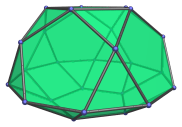The pentagonal orthocupolarotunda can be constructed by joining a pentagonal rotunda to a pentagonal cupola at their decagonal face, such that the triangular faces of the rotunda share edges with the square faces of the cupola. The ortho- in the name refers to how the orientation of the top and bottom pentagons are aligned with each other. Joining the cupola and rotunda in gyro orientation produces the pentagonal gyrocupolarotunda (J33) instead.

There is a hidden connection between the pentagonal orthocupolarotunda and the icosahedron: it is the Stott expansion of a particular partial faceting of the icosahedron according to one of its 5-fold axes of symmetry. The animation below shows this relationship.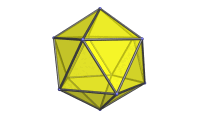This relationship causes the pentagonal orthocupolarotunda to show up as cells in a significant number of 4D CRF polytopes derived from the uniform polychora via a similar process.

## Projections

Here are some views of the pentagonal orthocupolarotunda from various angles:

Projection Description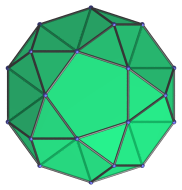Top view.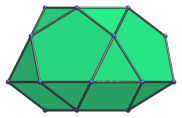Front view.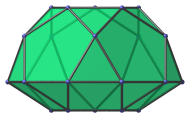Side view.

## Coordinates

The Cartesian coordinates of the pentagonal orthocupolarotunda with edge length 2 are:

• (−√((10+2√5)/5), 0, √((20+8√5)/5))
• (−√((5−√5)/10), ±φ, √((20+8√5)/5))
• ( √((5+2√5)/5), ±1, √((20+8√5)/5))
• ( √((20+8√5)/5), 0, √((10+2√5)/5))
• (−√((25+11√5)/10), ±φ, √((10+2√5)/5))
• ( √((5+√5)/10), ±φ2, √((10+2√5)/5))
• (±√(3+4φ), ±1, 0)
• (±√(2+φ), ±φ2, 0)
• (0, ±2φ, 0)
• (−√((10+2√5)/5), 0, −2√((3−φ)/5))
• (−√((5−√5)/10), ±φ, −2√((3−φ)/5))
• (√((5+2√5)/5), ±1, −2√((3−φ)/5))

where φ = (1+√5)/2 is the Golden Ratio, approximately 1.61803.

Last updated 02 Feb 2023.